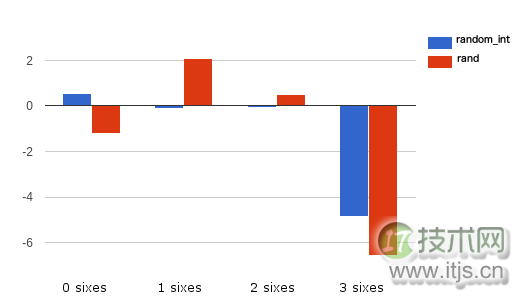# IT技术网www.itjs.cn# PHP中的随机性――你觉得自己幸运吗？CSPRNG可能主要用于:

## PHP7 中的CSPRNG

PHP 7引入了两个新函数可以用来实现CSPRNG： random_bytes 和 random_int。

random_bytes 函数返回一个字符串，接受一个int型入参代表返回结果的字节数。

```\$bytes = random_bytes('10');
var_dump(bin2hex(\$bytes));
//possible ouput: string(20) "7dfab0af960d359388e6"```

random_int 函数返回一个指定范围内的int型数字。

```var_dump(random_int(1, 100));
//possible output: 27```

## 一个简单的测试

0 个6 = 57.9 次 1 个6 = 34.7次 2 个6 = 6.9次 3 个6 = 0.5次

```\$times = 1000000;
\$result = [];
for (\$i=0; \$i<\$times; \$i++){
\$dieRoll = array(6 => 0); //initializes just the six counting to zero
\$dieRoll[roll()] += 1; //first die
\$dieRoll[roll()] += 1; //second die
\$dieRoll[roll()] += 1; //third die
\$result[\$dieRoll] += 1; //counts the sixes
}
function roll(){
return random_int(1,6);
}
var_dump(\$result);```

 Sixes expected random_int 0 579000 579430 1 347000 346927 2 69000 68985 3 5000 4658（接近0的值更好）

## PHP5 呢

`composer require paragonie/random_compat`
```require 'vendor/autoload.php';
\$string = random_bytes(32);
var_dump(bin2hex(\$string));
\$int = random_int(0,255);
var_dump(\$int);
// int(81)```

random_compat 库和PHP7使用不同的顺序:

```fread() /dev/urandom if available
mcrypt_create_iv(\$bytes, MCRYPT_CREATE_IV)
COM('CAPICOM.Utilities.1')->GetRandom()
openssl_random_pseudo_bytes()```

```\$passwordChar = '0123456789abcdefghijklmnopqrstuvwxyzABCDEFGHIJKLMNOPQRSTUVWXYZ';
for (\$i = 0; \$i < \$passwordLength; ++\$i) {
}